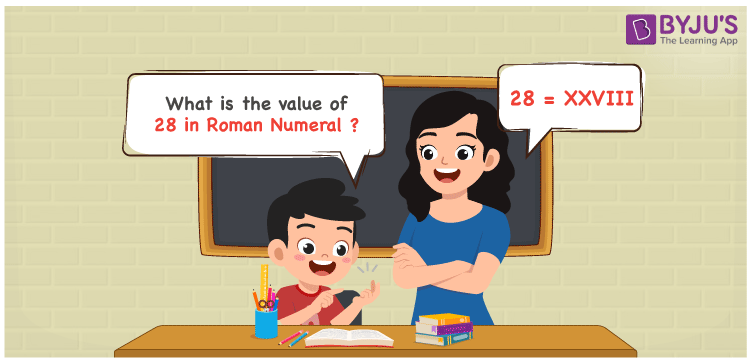# 28 in Roman Numerals

28 in Roman Numerals is XXVIII. To represent any number in Roman numerals, the 7 primary symbols are used with certain rules. Refer to the rules of Roman numerals. 28 is represented as XXVIII in the Roman numeral system. XXVIII follows the addition rule. In the representation XXVIII, the numerals X is added twice and the resultant is added to the sum of V and 3 I’s.

 Number Roman Numeral 28 XXVIII

## How to Write 28 in Roman Numerals?To convert 28 in Roman Numerals, we need to split the number according to the available symbols. i,e I, V, X, L, C, D, and M.

The simple way to split 28 will be 20 + 8. (But there are no primary letters for 20 and 8).

Hence 28 can be represented as 10 + 10 + 5 + 1 + 1 + 1.

28 = 10 + 10 + 5 + 1 + 1 + 1

28 = X + X + V + I + I + I

28 = XXVIII.

## Video Lesson on Roman Numerals## Frequently Asked Questions on 28 in Roman Numerals

Q1

### How to write the number 28 in Roman Numerals?

28 in Roman Numerals is written as XXVIII.
Q2

### Does XXI represent the number 28?

No. XXI represents the number 21.
Q3

### Does XXVIIIXXVIII represent a valid Roman numeral?

No. XXVIIIXXVIII does not represent the valid Roman numeral.